# Compact operator

(diff) ← Older revision | Latest revision (diff) | Newer revision → (diff)

An operatordefined on a subsetof a topological vector space with values in a topological vector space, such that every bounded subset ofis mapped by it into a pre-compact set (cf. Pre-compact space) of. If, in addition, the operatoris continuous on, then it is called completely continuous on this set. In the case whenandare Banach or, more generally, bornological spaces and the operator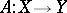is linear, the concept of a compact and a completely-continuous operator are the same. Ifis a compact andis a continuous operator, then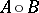and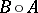are compact operators, so that the set of compact operators is a two-sided ideal in the ring of all continuous operators. In particular, a compact operator does not have a continuous inverse. The property of compactness plays an essential role in the theory of fixed points of an operator and in the study of its spectrum, which in this case has a number of "good" properties.

Examples of compact operators are the Fredholm integral operators (cf. Integral operator)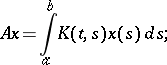the Hammerstein operator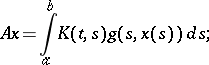and the Urysohn (Uryson) operator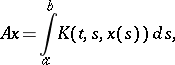in certain function spaces, under suitable restrictions on the functions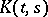,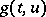and.

How to Cite This Entry:
Compact operator. Encyclopedia of Mathematics. URL: http://encyclopediaofmath.org/index.php?title=Compact_operator&oldid=13410
This article was adapted from an original article by V.I. Sobolev (originator), which appeared in Encyclopedia of Mathematics - ISBN 1402006098. See original article